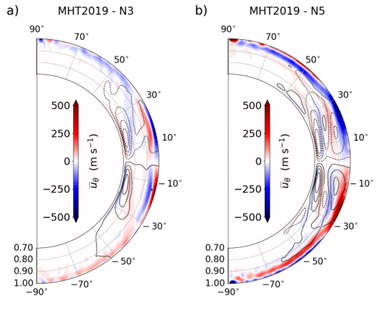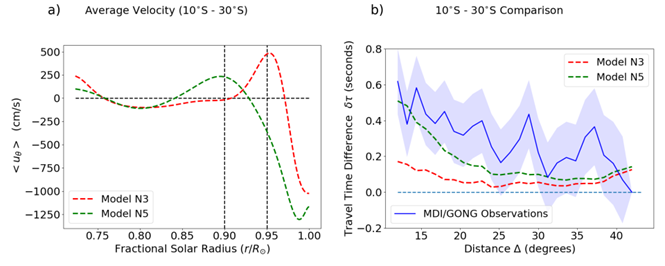# 188. Constraining Global Solar Models through Helioseismic Analysis

Contributed by Andrey Stejko. Posted on October 28, 2022

A. M. Stejko1, A. G. Kosovichev1, N. A. Featherstone2, G. Guerrero1,3, B. W. Hindman4,5, L. I. Matilsky4,5, and J. Warnecke6

1 New Jersey Institute of Technology, Newark, NJ 07012, USA
2 SWRI, Department of Space Studies, Boulder, CO 80302, USA
3 Universidade Federal de Minas Gerais, Belo Horizonte, MG, 31270-901, Brazil
4 JILA, University of Colorado, Boulder, CO 80309-0440, USA
5 Applied Mathematics, University of Colorado, Boulder, CO 80309-0526, USA
6 Max-Planck-Institut für Sonnensystemforschung, Justus-von-Liebig-Weg 3, D-37077 Göttingen, Germany

In order to develop a more robust understanding of global flow regimes in the solar interior, we apply a “forward-modeling” approach to the analysis of helioseismic signatures of meridional circulation profiles obtained from numerical simulations. We employ the global acoustic modeling code GALE to simulate the propagation of acoustic waves through regimes of mean mass-flows generated by global hydrodynamic and magnetohydrodynamic models: EULAG, the Pencil Code, and the Rayleigh code. These models are used to create synthetic Dopplergram data products, used as inputs for local time-distance helioseismology techniques. Helioseismic travel-time signatures from solutions obtained through global numerical simulations are then compared directly with inferences from solar observations, in order to set additional constraints on internal velocities in a direct way. This method allows us to better characterize the impact that known solutions have on helioseismic techniques directly, without the need to rely on subsequent inversions.Figure 1| Latitudinal velocities (uθ) amplified to a maximum of 500 m/s. Meridional circulation profiles are generated by the Rayleigh code, contrasting models with three density scale heights (a, N3) and five density scale heights (b, N5). Solid and dashed contours represent counterclockwise and clockwise circulation, respectively, with positive (negative) values depicting a southward (northwards) flow.

The analysis of two Rayleigh models in particular (N3 and N5) demonstrates the potential impact of a larger, more coherent, primary circulation cell on the model surface—generated as the result of a weaker near-surface rotational constraint. The meridional circulation profiles of these models can be seen in Fig. 1. The models are actuated with identical input parameters, with the exception of the number of density scale heights; Nρ = 3 for Model N3 and Nρ = 5 for Model N5, resulting in bulk Rossby numbers of Ro = 0.1345 and Ro = 0.4793, respectively, for a rotation rate of Ω0 = 3Ω, where Ω = 2.6 × 10−6 s−1. An analysis of the local Rossby numbers (defined as Ro = vrms(r)(2Ω0Hρ(r))−1, where vrms(r) is a spherically averaged RMS velocity and Hρ(r) is the local density scale height) in Ref.  shows that the increased near-surface stratification in Model N5 results in a rotationally unconstrained layer above r/ro ∼ 0.97, where ro = 6.586 × 1010 cm, in their model, and corresponds to the solar radius (ro = R) after we stretch it. Angular momentum transport in this region is dominated by inwardly directed turbulent Reynolds stresses resulting from the increased convective transport of downflow plumes. The impact of angular momentum transport due to columnar convection (Busse columns) is limited to rotationally constrained convection in the interior, allowing for the development of a larger more continuous global meridional flow cell near the surface of Model N5.Figure 2| a) The average latitudinal velocity θ> in the 10°S−30°S latitude range for Models N3 (red) and N5 (green), stretched to the solar surface (R). b) The N-S travel-time differences (δτNS) as a function of travel distance (Δ) for MDI/GONG data from Ref. . Latitude ranges in both hemispheres are averaged in order to reduce noise and are compared to dashed lines representing latitudinal averages for Models N3 (red) and N5 (green). The error range (blue region) is computed as the standard deviation of the travel-time differences in the 10°N − 10°S latitude range from zero.

The resulting formation of the internal meridional flow structure is illustrated by the average velocities in the 10°S−30°S latitudinal range for models N3 and N5 shown in panel (a) of Figure 2. Their contrasting impact on the helioseismic response can be seen in the measured N-S (North-South) travel-times (dashed lines) averaged over this latitudinal range, shown in panel (b), alongside their observational counterpart—computed in Ref.  (solid line). The travel-time differences calculated for Model N5 are a better match for the observational signal at depths r > 0.80R—exhibiting an average z-score of zN5 = 0.803, as opposed to zN3 = 1.598 for Model N3. Assuming that the travel-time difference measurements of Models N3 and N5 are the mean of a normal noise distribution, the probability of measuring a signal at least as extreme (p-value) as the travel-time differences computed by in Ref.  (10°S − 30°S, 12° < ∆ < 30°) would be approximately pN3 = 0.055 for Model N3 and pN5 = 0.21 for Model N5—representing a significantly increased likelihood that the average depth of the maximum return flow in this latitude range is approximately at or slightly below r ∼ 0.90R. This result, however, is strongly dependent on the proper estimate of meridional flow strength on the solar surface, which is variable, requires long temporal sampling windows to accurately gauge[4,5], and becomes unreliable at higher latitudes (±45°). This analysis demonstrates a “forward-modeling” methodology that is currently being applied to help set constraints on solar observations as well as to analyze the impact of known solutions on the idiosyncrasies of various helioseismic techniques.

### References:

 Stejko, A. M., Kosovichev, A. G., & Mansour, N. N. 2021, ApJS, 253, 9
 Matilsky, L. I., Hindman, B. W., & Toomre, J. 2019, ApJ, 871, 217
 Gizon, L., Cameron, R. H., Pourabdian, M., et al. 2020, Science, 368, 1469
 Kosovichev, A. G., & Zhao, J. 2016, in Lecture Notes in Physics, Berlin Springer Verlag
 Roudier, T., ˇSvanda, M., Ballot, J., Malherbe, J. M., & Rieutord, M. 2018, A&A, 611, A92